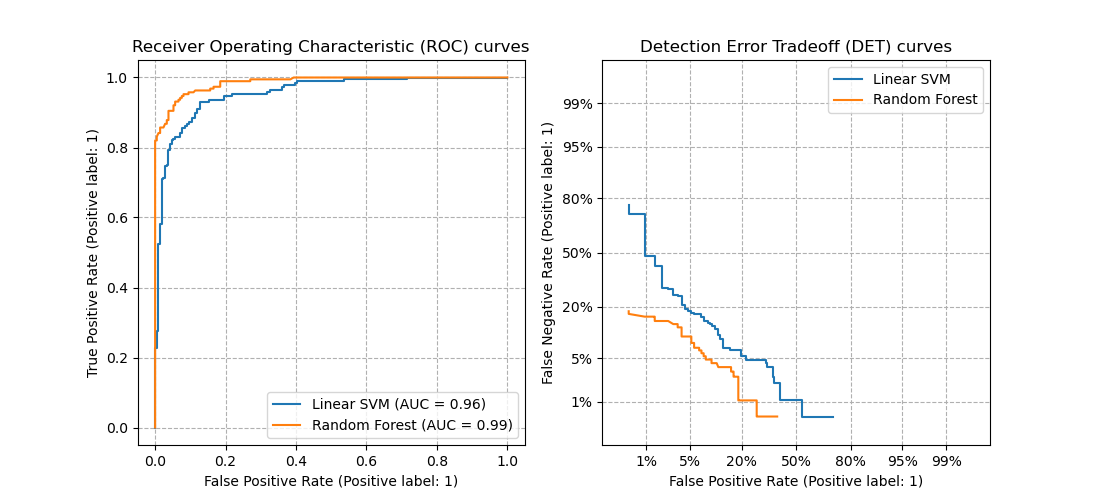# Detection error tradeoff (DET) curve¶

In this example, we compare receiver operating characteristic (ROC) and detection error tradeoff (DET) curves for different classification algorithms for the same classification task.

DET curves are commonly plotted in normal deviate scale. To achieve this plot_det_curve transforms the error rates as returned by the det_curve and the axis scale using scipy.stats.norm.

The point of this example is to demonstrate two properties of DET curves, namely:

1. It might be easier to visually assess the overall performance of different classification algorithms using DET curves over ROC curves. Due to the linear scale used for plotting ROC curves, different classifiers usually only differ in the top left corner of the graph and appear similar for a large part of the plot. On the other hand, because DET curves represent straight lines in normal deviate scale. As such, they tend to be distinguishable as a whole and the area of interest spans a large part of the plot.

2. DET curves give the user direct feedback of the detection error tradeoff to aid in operating point analysis. The user can deduct directly from the DET-curve plot at which rate false-negative error rate will improve when willing to accept an increase in false-positive error rate (or vice-versa).

The plots in this example compare ROC curves on the left side to corresponding DET curves on the right. There is no particular reason why these classifiers have been chosen for the example plot over other classifiers available in scikit-learn.

Noteimport matplotlib.pyplot as plt

from sklearn.datasets import make_classification
from sklearn.ensemble import RandomForestClassifier
from sklearn.metrics import plot_det_curve
from sklearn.metrics import plot_roc_curve
from sklearn.model_selection import train_test_split
from sklearn.pipeline import make_pipeline
from sklearn.preprocessing import StandardScaler
from sklearn.svm import LinearSVC

N_SAMPLES = 1000

classifiers = {
"Linear SVM": make_pipeline(StandardScaler(), LinearSVC(C=0.025)),
"Random Forest": RandomForestClassifier(
max_depth=5, n_estimators=10, max_features=1
),
}

X, y = make_classification(
n_samples=N_SAMPLES, n_features=2, n_redundant=0, n_informative=2,
random_state=1, n_clusters_per_class=1)

X_train, X_test, y_train, y_test = train_test_split(
X, y, test_size=.4, random_state=0)

# prepare plots
fig, [ax_roc, ax_det] = plt.subplots(1, 2, figsize=(11, 5))

for name, clf in classifiers.items():
clf.fit(X_train, y_train)

plot_roc_curve(clf, X_test, y_test, ax=ax_roc, name=name)
plot_det_curve(clf, X_test, y_test, ax=ax_det, name=name)